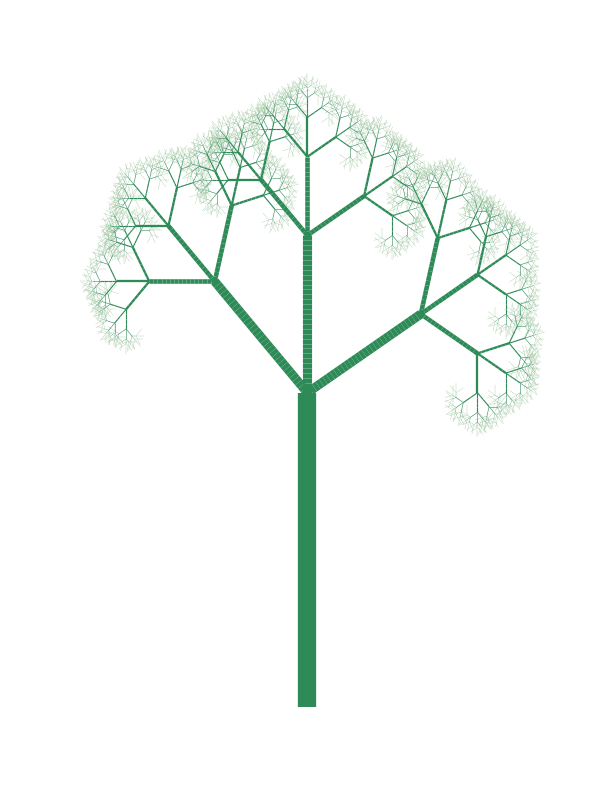L-system script that asks user for input parameters for a tree the L-system¶

In :
########################## IMPORTS ###############################

import os
import sys
import numpy as np
import re
import math
os.environ['PATH']

import matplotlib.pyplot as plt
from matplotlib import collections as mc
from matplotlib import colors
#from matplotlib import rc
#rc('font',**{'family':'sans-serif','sans-serif':['Helvetica']})
## for Palatino and other serif fonts use:
#rc('font',**{'family':'serif','serif':['Palatino']})
#rc('text', usetex=True)

##################################################################

In :
######################### FUNCTIONS ##############################
def L_system(axiom,rules,reps):
'''
L-system implementation function:
looks through every element in a string, starting with the axiom
and if a rule exists for that element, it is replaced with whatever the
rule maps it to.

args:
: axiom : type = str, starting point to build off of
: rules : type = dict, of the rules of the L-sys where key string elements when found
in an iteration, will be replaced with their value elements in the dict.
: reps : type = int, number of times to find and replace in the string
returns:
a dictionary of:
'str': the final axiom string
'rules': the original rules dictionary
'reps': the number of iterations done
'''
for i in range(reps):
for seed in sorted(rules.keys()):
axiom = re.sub(seed,rules[seed],axiom) #axiom.replace(seed,rules[seed])
print(list(axiom[:20]))
print('...')

return axiom

def Draw_Lsystem(final_str,rules,reps):
#drawing L-system:
print('The final L_system string is {} characters long...'.format(len(list(final_str))))
L_sys_string = final_str

draw_dim = {}
for key in rules.keys():
draw_dim[key] = float(input('How long should the lines be when drawing: "{}"? '.format(key)))
draw_dim['+'] = float(input('How many degrees should I turn to the left? '))* math.pi /180
draw_dim['-'] = -float(input('How many degrees should I turn to the right? '))* math.pi /180

# determine starting point and initial angle:
init_cond = (0,0,math.pi/2)

# initiate starting point and initial angle
x = init_cond
y = init_cond
ang = init_cond

stack = {'x':[x],'y':[y],'ang':[ang]}
cntr = 0

steps = list(L_sys_string)

lines = {dim:[] for dim in rules.keys()}
trunk = float(input('What thickness do you want to start with? (between 1 & {}) '.format(reps)))/reps
thickness = {dim:[] for dim in rules.keys()}
thkness_cntr = {dim:0 for dim in rules.keys()}
last_step = ''
rand_len = input('Would you like to randomize the length? (Y/N) ')
rand_ang = input('Would you like to randomize the angles? (Y/N) ')
turncount = 1

for i,step in enumerate(steps):
if (last_step != step) and last_step in thickness:
thickness[last_step].extend(thkness_cntr[last_step]*[thkness_cntr[last_step]*trunk])
thkness_cntr[last_step] = 0

if step in list(rules.keys()):
if rand_len == 'Y':
mult = np.absolute(np.random.normal(loc=0.0,scale=trunk/3))
else:
mult = 1
next_x = x + draw_dim[step] * mult * math.cos(ang)
next_y = y + draw_dim[step] * mult * math.sin(ang)
lines[step].append([[x,y],[next_x,next_y]])
x = next_x
y = next_y
thkness_cntr[step] += 1

elif step.isnumeric():
turncount = int(step)

elif step in ['+','-']:
if rand_ang == 'Y':
rang = np.random.normal(loc=0.0,scale=math.pi/12)
else:
rang = 0
for i in range(turncount):
ang = ang + draw_dim[step] + rang
turncount = 1

elif step == '[':
stack['x'].append(x)
stack['y'].append(y)
stack['ang'].append(ang)
cntr += 1

elif step == ']':
x = stack['x'][cntr]
y = stack['y'][cntr]
ang = stack['ang'][cntr]
stack['x'].pop(-1)
stack['y'].pop(-1)
stack['ang'].pop(-1)
cntr = cntr - 1

else:
print('error, unrecognized character!')

last_step = step
#if gif_it:
#plt.savefig('../assignments/assignment02-berenberg-howerter/figs/L-system_plots/gif-L-sys-{:02d}/step-{:02d}.pdf'.format(plot_counter,i),bbox_inches='tight')

c = ['darkseagreen','seagreen','lightseagreen','lightgreen']

fig, ax = plt.subplots(figsize=(10,14))

for i,dim in enumerate(lines.keys()):
lc = mc.LineCollection(lines[dim],colors=c[i],linewidths=thickness[dim])

ax.autoscale()
ax.margins(0.1)
plt.axis('off')
#plt.title(r'L-systems Plot - {}:{} - iterations = {}'.format(list(rules.keys()),list(rules.values()),reps),fontsize=15)

plt.show()

##################################################################

In :
print('********************** WELCOME **********************')

# option to use auto set-up:
YN = input('Would you like to build your own system (or use the auto-set-up)? (Y/N) ')

if YN == 'Y':
axiom = input('What is the axiom of your L-system? ')

rules = {}
key1 = str(input('What is the first character you want to make a rule for? '))
rules[key1] = str(input('What would you like "{}" to map to? '.format(key1)))
key2 = str(input('What is the second character you want to make a rule for? '))
rules[key2] = str(input('What would you like "{}" to map to? '.format(key2)))
more_rules = int(input('How many more rules do you want to add?'))
for i in range(more_rules):
k = str(input('What is the next character you want to make a rule for? '))
rules[k] = str(input('What would you like "{}" to map to? '.format(k)))
else:
axiom = 'G'
rules = {'G':'F[+G][-G]G','F':'FF'}

# ask for number of iterations:
reps = int(input('How many iterations of the L-system would you like to perform? (recommend only up to 10) '))
if reps == '':
reps = 5

# call L-system build script:
print('Building L-system...')
final_str = L_system(axiom,rules,reps)

# call L-system plotting script:
print('Drawing L-system...')
Draw_Lsystem(final_str,rules,reps)

********************** WELCOME **********************
Would you like to build your own system (or use the auto-set-up)? (Y/N) N
How many iterations of the L-system would you like to perform? (recommend only up to 10) 7
Building L-system...
['F', '[', '+', 'G', ']', '[', '-', 'G', ']', 'G']
...
['F', 'F', '[', '+', 'F', '[', '+', 'G', ']', '[', '-', 'G', ']', 'G', ']', '[', '-', 'F', '[', '+']
...
['F', 'F', 'F', 'F', '[', '+', 'F', 'F', '[', '+', 'F', '[', '+', 'G', ']', '[', '-', 'G', ']', 'G']
...
['F', 'F', 'F', 'F', 'F', 'F', 'F', 'F', '[', '+', 'F', 'F', 'F', 'F', '[', '+', 'F', 'F', '[', '+']
...
['F', 'F', 'F', 'F', 'F', 'F', 'F', 'F', 'F', 'F', 'F', 'F', 'F', 'F', 'F', 'F', '[', '+', 'F', 'F']
...
['F', 'F', 'F', 'F', 'F', 'F', 'F', 'F', 'F', 'F', 'F', 'F', 'F', 'F', 'F', 'F', 'F', 'F', 'F', 'F']
...
['F', 'F', 'F', 'F', 'F', 'F', 'F', 'F', 'F', 'F', 'F', 'F', 'F', 'F', 'F', 'F', 'F', 'F', 'F', 'F']
...
Drawing L-system...
The final L_system string is 10804 characters long...
How long should the lines be when drawing: "G"? 2
How long should the lines be when drawing: "F"? 1
How many degrees should I turn to the left? 45
How many degrees should I turn to the right? 60
What thickness do you want to start with? (between 1 & 7) 2
Would you like to randomize the length? (Y/N) y
Would you like to randomize the angles? (Y/N) yIn [ ]: